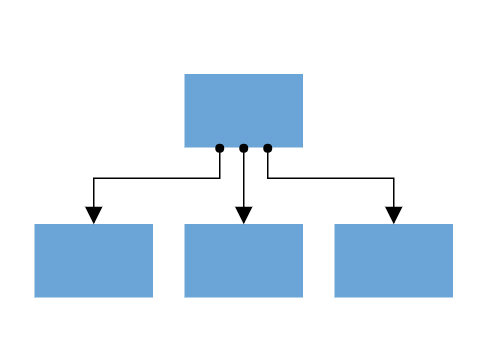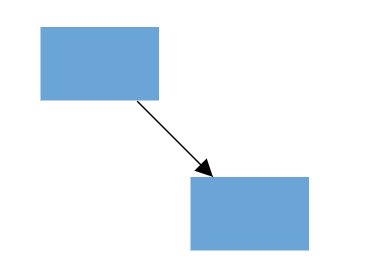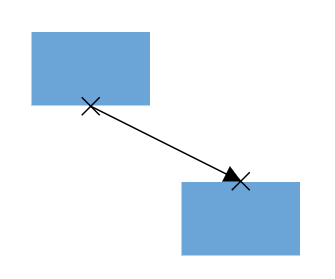Search results

# Port in ASP.NET Core Diagram control

22 Jul 2021 / 3 minutes to read

Diagram provides support to define custom ports for making connections.When a connector is connected between two nodes, its end points are automatically docked to the node’s nearest boundary as shown in the following image.Ports act as the connection points of the node and allows to create connections with only those specific points as shown in the following image.## Add ports when initializing nodes

To add a connection port, define the port object and add it to node’s ports collection. The `offset` property of port accepts an object of fractions and used to determine the position of ports. The following code illustrates how to add ports when initializing the node.

tagHelper
port.cs
``````<ejs-diagram id="container" width="100%" height="700px" nodes="@ViewBag.nodes">
</ejs-diagram>``````
``````using System;
using System.Collections.Generic;
using System.Linq;
using System.Web;
using System.Web.Mvc;
using Syncfusion.EJ2.Diagrams;
using System.Drawing;

namespace EJ2MVCSampleBrowser.Controllers.Diagram {
public partial class DiagramController: Controller {
// GET: Nodes
public ActionResult Nodes() {

List < DiagramPort > ports1 = new List < DiagramPort > ();
Id = "port1", Shape = PortShapes.Circle,
Offset = new DiagramPoint() {
X = 0.5, Y = 0.5
}
});
List < DiagramNode > nodes = new List < DiagramNode > ();
Id = "node1",
Width = 100,
Height = 100,
Style = new NodeStyleNodes() {
Fill = "#6BA5D7",
StrokeColor = "White"
},
text = "node1",
OffsetX = 100,
OffsetY = 100,
Ports = ports1
});
ViewBag.nodes = nodes;

return View();
}
}
public class Node: DiagramNode {
public string text;
}
}``````

Add ports at runtime by using the client-side method `addPorts`. The following code illustrates how to add ports to node at runtime.

The port’s ID property is used to define the unique ID for the port and its further used to find the port at runtime. If ID is not set, then default ID is automatically set.

tagHelper
run.cs
``````<ejs-diagram id="container" width="100%" height="700px" nodes="@ViewBag.nodes">
</ejs-diagram>``````
``````using System;
using System.Collections.Generic;
using System.Linq;
using System.Web;
using System.Web.Mvc;
using Syncfusion.EJ2.Diagrams;
using System.Drawing;

namespace EJ2MVCSampleBrowser.Controllers.Diagram {
public partial class DiagramController: Controller {
// GET: Nodes
public ActionResult Nodes() {
List < DiagramNode > nodes = new List < DiagramNode > ();
Id = "node1",
Width = 100,
Height = 100,
Style = new NodeStyleNodes() {
Fill = "#6BA5D7",
StrokeColor = "White"
},
text = "node1",
OffsetX = 100,
OffsetY = 100,
});
ViewBag.nodes = nodes;

return View();
}
}
public class Node: DiagramNode {
public string text;
}
}``````
``````// Initialize port collection
var port= [{
id: 'port1',
offset: {
x: 0,
y: 0.5
},
visibility: PortVisibility.Visible
} {
id: 'port2',
offset: {
x: 1,
y: 0.5
},
visibility: PortVisibility.Visible
},
{
id: 'port3',
offset: {
x: 0.5,
y: 0
},
visibility: PortVisibility.Visible
},
{
id: 'port4',
offset: {
x: 0.5,
y: 1
},
visibility: PortVisibility.Visible
}
];

// Method to add ports through run time

## Remove ports at runtime

Remove ports at runtime by using client-side method `removePorts`. Refer to the following example which shows how to remove ports at runtime.

tagHelper
remove.cs
``````<ejs-diagram id="container" width="100%" height="700px" nodes="@ViewBag.nodes">
</ejs-diagram>``````
``````using System;
using System.Collections.Generic;
using System.Linq;
using System.Web;
using System.Web.Mvc;
using Syncfusion.EJ2.Diagrams;
using System.Drawing;

namespace EJ2MVCSampleBrowser.Controllers.Diagram {
public partial class DiagramController: Controller {
// GET: Nodes
public ActionResult Nodes() {
List < DiagramPort > ports1 = new List < DiagramPort > ();
Id = "port1", Shape = PortShapes.Circle, Offset = new DiagramPoint() {
X = 0, Y = 0.5
}, Visibility = PortVisibility.Visible
});
Id = "port2", Shape = PortShapes.Circle, Offset = new DiagramPoint() {
X = 1, Y = 0.5
}, Visibility = PortVisibility.Visible
});
Id = "port3", Shape = PortShapes.Circle, Offset = new DiagramPoint() {
X = 0.5, Y = 1
}, Visibility = PortVisibility.Visible
});
Id = "port4", Shape = PortShapes.Circle, Offset = new DiagramPoint() {
X = 1, Y = 1
}, Visibility = PortVisibility.Visible
});

List < DiagramNode > nodes = new List < DiagramNode > ();
Id = "node1",
Width = 100,
Height = 100,
Style = new NodeStyleNodes() {
Fill = "#6BA5D7",
StrokeColor = "White"
},
text = "node1",
OffsetX = 100,
OffsetY = 100,
Ports = ports1
});
ViewBag.nodes = nodes;

return View();
}
}
public class Node: DiagramNode {
public string text;
}
}``````
``````var ports = [{
id: 'port1',
}, {
id: 'port2',
}, {
id: 'port3',
}, {
id: 'port4',
}]
diagram.removePorts(diagram.nodes, ports);``````

## Update port at runtime

You can change any port properties at runtime and update it through the client-side method `dataBind`.

The following code example illustrates how to change the port properties.

tagHelper
port.cs
``````<ejs-diagram id="container" width="100%" height="700px" nodes="@ViewBag.nodes">
</ejs-diagram>``````
``````using System;
using System.Collections.Generic;
using System.Linq;
using System.Web;
using System.Web.Mvc;
using Syncfusion.EJ2.Diagrams;
using System.Drawing;

namespace EJ2MVCSampleBrowser.Controllers.Diagram {
public partial class DiagramController: Controller {
// GET: Nodes
public ActionResult Nodes() {

List < DiagramPort > ports1 = new List < DiagramPort > ();
Id = "port1", Shape = PortShapes.Circle,
Offset = new DiagramPoint() {
X = 0.5, Y = 0.5
}
});
List < DiagramNode > nodes = new List < DiagramNode > ();
Id = "node1",
Width = 100,
Height = 100,
Style = new NodeStyleNodes() {
Fill = "#6BA5D7",
StrokeColor = "White"
},
text = "node1",
OffsetX = 100,
OffsetY = 100,
Ports = ports1
});
ViewBag.nodes = nodes;

return View();
}
}
public class Node: DiagramNode {
public string text;
}
}``````
``````diagram.nodes.ports.offset = {
x: 1,
y: 1
};
diagram.dataBind();``````

## Appearance

The following code illustrates how to change the appearance of port.

tagHelper
``````<ejs-diagram id="container" width="100%" height="700px" nodes="@ViewBag.nodes">
</ejs-diagram>``````

## Offset

The offset property of port is used to align the port based on fractions. 0 represents top/left corner, 1 represents bottom/right corner, and 0.5 represents half of width/height.

## Constraints

The constraints property allows to enable/disable certain behaviors of ports. For more information about port constraints, refer to `Port Constraints`.